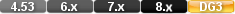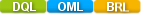Easy to Create, Easy to Change - Easy to use!## Cos (cosine)cos(numeric field) cos(numeric value) cos(90)

The cos function calculates the cosine of an angle expressed in radians. The value returned ranges between -1 and 1.

# Parameters

Numeric value expressing an angle in Radians.

# Returns/Result

Numerical value between -1 via 0 to +1

# Examples

Examples 1

cos( 3.1415928)

Returns: -1

Examples 2

cos( -22)

Returns: .9271838

# cos (cosine)

#### Type

Trigonometric Function

#### Purpose

The cos function calculates the cosine of an angle expressed in radians. The value returned ranges between -1 and 1.

Syntax

cos( NUMERIC VALUE)

Returns

A numeric value.

Usage

The numeric value in a Trigonometric function can be a constant value (as shown below), a variable, a field value or an expression.

Examples

cos( 3.1415928)

Returns: -1

cos( -22)

Returns: .9271838